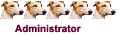## all kinds of beauties of Mathematics

### all kinds of beauties of Mathematics

https://cartesianproduct.wordpress.com/ ... r-11-sets/Ask most people about set theory and you will get a blank look, but ask them about a Venn diagram and they are much more likely to understand: indeed Venn diagrams are so well grasped that Mitt Romney’s campaign for the US Presidency recently attempted to make use of them (though I am not sure it was much of a success, but that’s another story…)

So called 2-Venn (two circles) and 3-Venn diagrams are very familiar. But higher dimension Venn diagrams that are (relatively) easy to grasp (I’ll explain what I mean by that below) are actually difficult to produce – and until last month nobody had managed to get beyond 7.

3-Venn diagram

So, let’s state a few basic properties of any Venn diagram (here is a good general survey of Venn diagrams)- firstly – each region (face) is unique – there is only one region where the bottom curve intersects with the right curve alone, and only one where it intersects with the left curve alone and only one where all three curves intersect (the grey region) and so on.

This image (taken from that survey, apologies) – shows a series of set intersections which are not a Venn diagram:

Not a Venn diagramFor instance, we can see the two shaded areas both represent intersections of the ‘blue’ and ‘red’ sets.

A second point is that there is a finite number of intersections. In other words segments of curves cannot lie on top of one another (in fact this rule means the intersections must be in the form of Eulerian points of zero length – as, following on from the last post about Aristotle’s Wheel Paradox, any segment of a curve is continuous and has an uncountable infinite number of points).
The 3-Venn example above illustrates some of the key points about easier to understand Venn diagrams – firstly it is simple: no intersection is of more than two curves and secondly it is symmetric. In fact, if we are willing to ignore these points we can draw Venn diagrams of any number of sets, each with less intelligibility than the last.

Drawing higher number simple and symmetric Venn diagrams is exceptionally difficult and it has been proved that such n-Venn diagrams only exist when n is a prime.

So we have 2-Venns and 3-Venns, and mathematicians have managed 5-Venns:Simple, symetric 5-Venn from Journal of CombinatronicsAnd 7-Venns:

Simple, symmetric 7-Venn from Journal of Combinatronics

But, until now, simple symmetric 11-Venns have been elusive. Certainly 11-Venn’s have been around – as the example below shows:

Symmetric, non-simple 11-Venn from Journal of CombinatronicsThis example is symmetric but it is not simple.

Now, though, a breakthrough has been made. Named newroz – the Kurdish name for the new year – the first simple, symmetric 11-Venn has come from Khalegh Mamakani and Frank Ruskey, both of the Department of Computer Science at the University of Victoria, Canada.
• 0

 Share/分享:think unique,be special